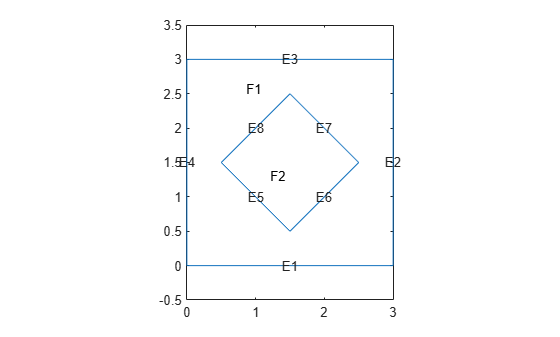# ModalThermalResults

Modal thermal solution

## Description

A `ModalThermalResults` object contains the eigenvalues and eigenvector matrix of a thermal model, and average of snapshots used for proper orthogonal decomposition (POD).

## Creation

Solve a modal thermal problem using the `solve` function. This function returns a modal thermal solution as a `ModalThermalResults` object.

## Properties

expand all

Eigenvalues of a thermal model, returned as a column vector.

Data Types: `double`

Eigenvector matrix, returned as a matrix.

Data Types: `double`

Average of snapshots used for POD, returned as a column vector.

Data Types: `double`

Type of modes, returned as `"EigenModes"` or `"PODModes"`.

Data Types: `string`

Finite element mesh, returned as an `FEMesh` object. For details, see FEMesh Properties.

## Examples

collapse all

Solve a transient thermal problem by first obtaining mode shapes for a particular decay range and then using the modal superposition method.

Modal Decomposition

First, create a modal thermal model.

`thermalmodel = createpde("thermal","modal");`

Create the geometry and include it in the model.

```SQ1 = [3; 4; 0; 3; 3; 0; 0; 0; 3; 3]; D1 = [2; 4; 0.5; 1.5; 2.5; 1.5; 1.5; 0.5; 1.5; 2.5]; gd = [SQ1 D1]; sf = 'SQ1+D1'; ns = char('SQ1','D1'); ns = ns'; dl = decsg(gd,sf,ns); geometryFromEdges(thermalmodel,dl); pdegplot(thermalmodel,"EdgeLabels","on","FaceLabels","on") xlim([-1.5 4.5]) ylim([-0.5 3.5]) axis equal```For the square region, assign these thermal properties:

• Thermal conductivity is $10\text{\hspace{0.17em}}\mathrm{W}/\left(\mathrm{m}{\cdot }^{\circ }\mathrm{C}\right)$.

• Mass density is $2\text{\hspace{0.17em}}\mathrm{kg}/{\mathrm{m}}^{3}$.

• Specific heat is $0.1\text{\hspace{0.17em}}\mathrm{J}/\left({\mathrm{kg}\cdot }^{\circ }\mathrm{C}\right)$.

```thermalProperties(thermalmodel,"ThermalConductivity",10, ... "MassDensity",2, ... "SpecificHeat",0.1, ... "Face",1);```

For the diamond region, assign these thermal properties:

• Thermal conductivity is $2\text{\hspace{0.17em}}\mathrm{W}/\left(\mathrm{m}{\cdot }^{\circ }\mathrm{C}\right)$.

• Mass density is $1\text{\hspace{0.17em}}\mathrm{kg}/{\mathrm{m}}^{3}$.

• Specific heat is $0.1\text{\hspace{0.17em}}\mathrm{J}/\left({\mathrm{kg}\cdot }^{\circ }\mathrm{C}\right)$.

```thermalProperties(thermalmodel,"ThermalConductivity",2, ... "MassDensity",1, ... "SpecificHeat",0.1, ... "Face",2);```

Assume that the diamond-shaped region is a heat source with a density of $4\text{\hspace{0.17em}}\mathrm{W}/{\mathrm{m}}^{2}$.

`internalHeatSource(thermalmodel,4,"Face",2);`

Apply a constant temperature of 0 °C to the sides of the square plate.

`thermalBC(thermalmodel,"Temperature",0,"Edge",[1 2 7 8]);`

Set the initial temperature to 0 °C.

`thermalIC(thermalmodel,0);`

Generate the mesh.

`generateMesh(thermalmodel);`

Compute eigenmodes of the thermal model in the decay range [100,10000] ${s}^{-1}$.

`RModal = solve(thermalmodel,"DecayRange",[100,10000])`
```RModal = ModalThermalResults with properties: DecayRates: [164x1 double] ModeShapes: [1481x164 double] ModeType: "EigenModes" Mesh: [1x1 FEMesh] ```

Transient Analysis

Knowing the mode shapes, you can now use the modal superposition method to solve the transient thermal problem. First, switch the thermal model analysis type to transient.

`thermalmodel.AnalysisType = "transient";`

The dynamics for this problem are very fast. The temperature reaches a steady state in about 0.1 second. To capture the most active part of the dynamics, set the solution time to `logspace(-2,-1,100)`. This command returns 100 logarithmically spaced solution times between 0.01 and 0.1.

`tlist = logspace(-2,-1,10);`

Solve the equation.

`Rtransient = solve(thermalmodel,tlist,"ModalResults",RModal);`

Plot the solution with isothermal lines by using a contour plot.

```T = Rtransient.Temperature; pdeplot(thermalmodel,"XYData",T(:,end), ... "Contour","on", ... "ColorMap","hot")```Obtain POD modes of a linear thermal model using several instances of the transient solution (snapshots).

Create a transient thermal model.

`thermalmodel = createpde("thermal","transient");`

Create a unit square geometry and include it in the model.

`geometryFromEdges(thermalmodel,@squareg);`

Plot the geometry, displaying edge labels.

```pdegplot(thermalmodel,"EdgeLabels","on") xlim([-1.1 1.1]) ylim([-1.1 1.1])```Specify the thermal conductivity, mass density, and specific heat of the material.

```thermalProperties(thermalmodel,"ThermalConductivity",400, ... "MassDensity",1300, ... "SpecificHeat",600);```

Set the temperature on the right edge to `100`.

`thermalBC(thermalmodel,"Edge",2,"Temperature",100);`

Set an initial value of `0` for the temperature.

`thermalIC(thermalmodel,0);`

Generate a mesh.

`generateMesh(thermalmodel);`

Solve the model for three different values of heat source and collect snapshots.

```tlist = 0:10:600; snapShotIDs = [1:10 59 60 61]; Tmatrix = []; heatVariation = [10000 15000 20000]; for q = heatVariation internalHeatSource(thermalmodel,q); results = solve(thermalmodel,tlist); Tmatrix = [Tmatrix,results.Temperature(:,snapShotIDs)]; end```

Switch the thermal model analysis type to modal.

`thermalmodel.AnalysisType = "modal";`

Compute the POD modes.

`RModal = solve(thermalmodel,"Snapshots",Tmatrix)`
```RModal = ModalThermalResults with properties: DecayRates: [6x1 double] ModeShapes: [1541x6 double] SnapshotsAverage: [1541x1 double] ModeType: "PODModes" Mesh: [1x1 FEMesh] ```

## Version History

Introduced in R2022a